NetworkX

#### Previous topic

degree_centrality

Blockmodeling

# betweenness_centrality¶

betweenness_centrality(G, nodes)[source]

Compute betweenness centrality for nodes in a bipartite network.

Betweenness centrality of a node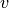is the sum of the fraction of all-pairs shortest paths that pass through.

Values of betweenness are normalized by the maximum possible value which for bipartite graphs is limited by the relative size of the two node sets [R118].

Let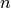be the number of nodes in the node set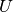and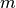be the number of nodes in the node set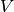, then nodes inare normalized by dividing by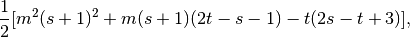where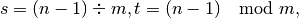and nodes inare normalized by dividing by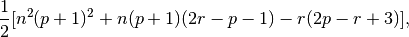where,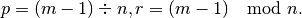Parameters : G : graph A bipartite graph nodes : list or container Container with all nodes in one bipartite node set. betweenness : dictionary Dictionary keyed by node with bipartite betweenness centrality as the value.